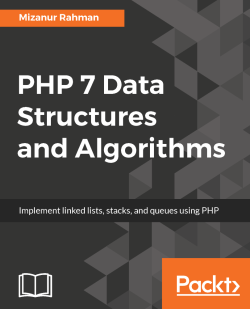# PHP 7 Data Structures and Algorithms5 (7 reviews total)
By Mizanur Rahman
• FREE Subscription Access now
• \$10.00 Was 35.99 eBook
What do you get with a Packt Subscription?

• Constantly updated with 100+ new titles each month
• Breadth and depth in over 1,000+ technologies

PHP has always been the the go-to language for web based application development, but there are materials and resources you can refer to to see how it works. Data structures and algorithms help you to code and execute them effectively, cutting down on processing time significantly.

If you want to explore data structures and algorithms in a practical way with real-life projects, then this book is for you.

The book begins by introducing you to data structures and algorithms and how to solve a problem from beginning to end using them. Once you are well aware of the basics, it covers the core aspects like arrays, listed lists, stacks and queues. It will take you through several methods of finding efficient algorithms and show you which ones you should implement in each scenario. In addition to this, you will explore the possibilities of functional data structures using PHP and go through advanced algorithms and graphs as well as dynamic programming.

By the end, you will be confident enough to tackle both basic and advanced data structures, understand how they work, and know when to use them in your day-to-day work

Publication date:
May 2017
Publisher
Packt
Pages
340
ISBN
9781786463890

# Introduction to Data Structures and Algorithms

We are living in a digital era. In every segment of our life and daily needs, we have a significant use of technology. Without technology, the world will virtually stand still. Have you ever tried to find what it takes to prepare a simple weather forecast? Lots of data are analyzed to prepare simple information, which is delivered to us in real time. Computers are the most important find of the technology revolution and they have changed the world drastically in the last few decades. Computers process these large sets of data and helps us in every technology-dependent task and need. In order to make computer operation efficient, we represent data in different formats or we can call in different structures, which are known as data structures.

Data structures are very important components for computers and programming languages. Along with data structures, it is also very important to know how to solve a problem or find a solution using these data structures. From our simple mobile phone contact book to complex DNA profile matching systems, the use of data structures and algorithms is everywhere.

Have we ever thought that standing in a superstore queue to payout can be a representation of data structure? Or taking out a bill from a pile of papers can be another use of data structure? In fact, we are following data structure concepts almost everywhere in our lives. Whether we are managing the queue to pay the bill or to get to the transportation, or maintaining a stack for a pile of books or papers for daily works, data structures are everywhere and impacting our lives.

PHP is a very popular scripting language and billions of websites and applications are built using it. People use Hypertext Preprocessor (PHP) for simple applications to very complex ones and some are very data intensive. The big question is--should we use PHP for any data intensive application or algorithmic solutions? Of course we should. With the new release of PHP 7, PHP has entered into new possibilities of efficient and robust application development. Our mission will be to show and prepare ourselves to understand the power of data structures and algorithms using PHP 7, so that we can utilize it in our applications and programs.

# Importance of data structures and algorithms

If we consider our real-life situation with computers, we also use different sorts of arrangements of our belongings and data so that we can use them efficiently or find them easily when needed. What if we enter our phone contact book in a random order? Will we be able to find a contact easily? We might end up searching each and every contact in the book as the contacts are not arranged in a particular order. Just consider the following two images:One shows that the books are scattered and finding a particular book will take time as the books are not organized. The other one shows that the books are organized in a stack. Not only does the second image show that we are using the space smartly, but also the searching of books becomes easier.

Let us consider another example. We are going to buy tickets for an important football match. There are thousands of people waiting for the ticket booth to open. Tickets are going to be distributed on a first come first served basis. If we consider the following two images, which one is the best way of handling such a big crowd?:The left image clearly shows that there is no proper order and there is no way to know who came first to get the tickets. But if we knew that people were waiting in a structured way, in a line, or queue, then it will be easier to handle the crowd and we will hand over the tickets to whoever came first. This is a common phenomenon known as a queue which is heavily used in the programming world. Programming terms are not generated from outside the world. In fact, the majority of the data structures are inspired from real life and they use the same terms most of the times. Whether we are preparing our task list, contact list, book piles, diet charting, preparing a family tree, or organization hierarchy, we are basically using different arrangement techniques which are known as data structures in the computing world.

We have talked a little about data structures so far but what about algorithms? Don't we use any algorithms in our daily lives? Definitely we do. Whenever we are searching for a contact from our old phone book, we are definitely not searching from the beginning. If we are searching for Tom, we will not search the page where it says A, B, or C. We are directly going to the page T and will find if Tom is listed there or not. Or, if we need to find a doctor from a telephone directory, we will definitely not search in the foods section. If we consider the phone book or telephone directory as data structures, then the way we search for particular information is known as algorithms. While data structures help us to use data efficiently, algorithms help us to perform different operations on those data efficiently. For example, if we have 100,000 entries in our phone directory, searching a particular entry from the beginning might take a long time. But, if we know the doctors are listed from page 200 to 220, we can search only those pages to save our time by searching a small section rather than the full directory:We can also consider a different way of searching for a doctor. While the previous paragraph takes the approach of searching a particular section of the directory, we can even search alphabetically within the directory, like the way we search a dictionary for a word. That might even reduce the time and entries for our searching. There can be many different approaches to find solutions of a problem, and each of the approaches can be named as algorithms. From the earlier discussion we can say that for a particular problem or task, there can be multiple ways or algorithms to perform. Then which one should we consider to use? We are going to discuss that very soon. Before moving to that point, we are going to focus on PHP data types and Abstract Data Types (ADT). In order to grasp the data structure concept, we must have a strong understanding of PHP data types and ADT.

# Understanding Abstract Data Type (ADT)

PHP has eight primitive data types and those are booleans, integer, float, string, array, object, resource, and null. Also, we have to remember that PHP is a weakly typed language and that we are not bothered about the data type declaration while creating those. Though PHP has some static type features, PHP is predominantly a dynamically typed language which means variables are not required to be declared before using it. We can assign a value to a new variable and use it instantly.

For the examples of data structures we have discussed so far can we use any of the primitive data types to represent those structures? Maybe we can or maybe not. Our primitive data types have one particular objective: storing data. In order to achieve some flexibility in performing operations on those data, we will require using the data types in such a way so that we can use them as a particular model and perform some operations. This particular way of handling data through a conceptual model is known as Abstract Data Type, or ADT. ADT also defines a set of possible operations for the data.

We need to understand that ADTs are mainly theoretical concepts which are used in design and analysis of algorithms, data structures, and software design. In contrast, data structures are concrete representations. In order to implement an ADT, we might need to use data types or data structures or both. The most common example of ADTs is stack and queue: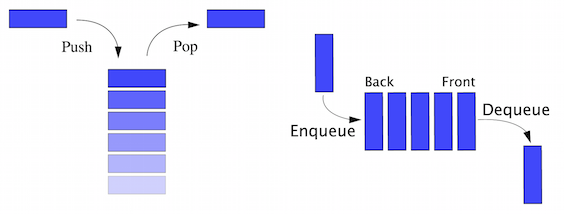Considering the stack as ADT, it is not only a collection of data but also two important operations called push and pop. Usually, we put a new entry at the top of the stack which is known as push and when we want to take an item, we take from the top which is also known as pop. If we consider PHP array as a stack, we will require additional functionality to achieve these push and pop operations to consider it as stack ADT. Similarly, a queue is also an ADT with two required operations: to add an item at the end of the queue also known as enqueue and remove an item from the beginning of the queue, also known as dequeue. Both sound similar but if we give a close observation we will see that a stack works as a Last-In, First-Out (LIFO) model whereas a queue works as a First-In, First-Out (FIFO) model. These two different mathematical models make them two different ADTs.

• List
• Map
• Set
• Stack
• Queue
• Priority queue
• Graph
• Tree

In coming chapters, we will explore more ADTs and implement them as data structures using PHP.

# Different data structures

We can categorize data structures in to two different groups:

• Linear data structures
• Nonlinear data structures

In linear data structures, items are structured in a linear or sequential manner. Array, list, stack, and queue are examples of linear structures. In nonlinear structures, data are not structured in a sequential way. Graph and tree are the most common examples of nonlinear data structures.

Let us now explore the world of data structures, with different types of data structures and their purposes in a summarized way. Later on, we will explore each of the data structures in details.

There are many different types of data structures that exist in the programming world. Out of them, following are the most used ones:

• Struct
• Array
• Stack
• Queue
• Priority queue
• Set
• Map
• Tree
• Graph
• Heap

# Struct

Usually, a variable can store a single data type and a single scalar data type can only store a single value. There are many situations where we might need to group some data types together as a single complex data type. For example, we want to store some student information together in a student data type. We need the student name, address, phone number, email, date of birth, current class, and so on. In order to store each student record to a unique student data type, we will need a special structure which will allow us to do that. This can be easily achieved by struct. In other words, a struct is a container of values which is typically accessed using names. Though structs are very popular in C programming language, we can use a similar concept in PHP as well. We are going to explore that in coming chapters.

# Array

Though an array is considered to be a data type in PHP, an array is actually a data structure which is mostly used in all programming platforms. In PHP, the array is actually an ordered map (we are going to know about maps after a few more sections). We can store multiple values in a single array as a single variable. Matrix type data are easy to store in an array and hence it is used widely in all programming platforms. Usually arrays are a fixed size collection which is accessed by sequential numeric indexes. In PHP, arrays are implemented differently and you can define dynamic arrays without defining any fixed size of the array. We will explore more about PHP arrays in the next chapter. Arrays can have different dimensions. If an array has only one index to access an element, we call it a single dimension array. But if it requires two or more indexes to access an element, we call it two dimensional or multidimensional arrays respectively. Here are two diagrams of array data structures: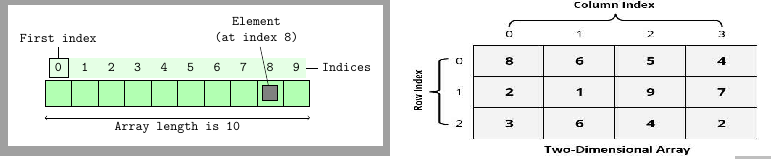A linked list is a linear data structure which is a collection of data elements also known as nodes and can have varying sizes. Usually, listed items are connected through a pointer which is known as a link and hence it is known as a linked list. In a linked list, one list element links to the next element through a pointer. From the following diagram, we can see that the linked list actually maintains an ordered collection. Linked lists are the most common and simplest form of data structures used by programming languages. In a single linked list, we can only go forward. In Chapter 3, Using Linked Lists we are going to dive deep inside the linked list concepts and implementations: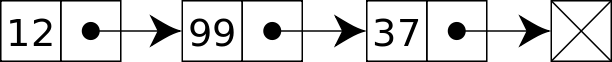A doubly linked list is a special type of linked list where we not only store what is the next node, but we also store the previous node inside the node structure. As a result, it can move forward and backward within the list. It gives more flexibility than a single linked list or linked list by having both the previous and next pointers. We are going to explore more about these in Chapter 3, Using Linked Lists. The following diagram depicts a doubly linked list: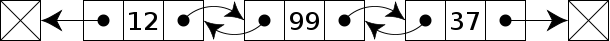# Stack

As we talked about the stack in previous pages, we already know that stack is a linear data structure with the LIFO principle. As a result, stacks have only one end to add a new item or remove an item. It is one of the oldest and most used data structures in computer technology. We always add or remove an item from a stack using the single point named top. The term push is used to indicate an item to be added on top of the stack and pop to remove an item from the top; this is shown in the following diagram. We will discuss more about stacks in Chapter 4, Constructing Stacks and Queues.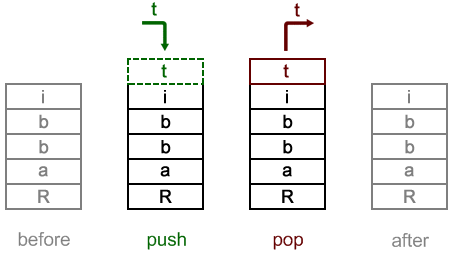# Queue

A queue is another linear data structure which follows the FIFO principle. A queue allows two basic operations on the collection. The first one is enqueue which allows us to add an item to the back of the queue. The second one is dequeue which allows us to remove an item from the front of the queue. A queue is another of the most used data structures in computer technology. We will learn details about queues in Chapter 4, Consrtucting Stacks and Queues.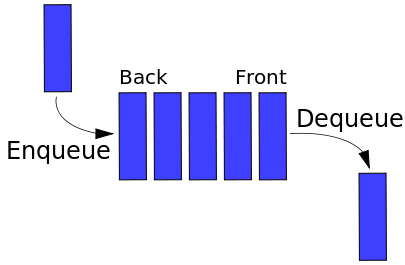# Set

A set is an abstract data type which is used to store certain values. These values are not stored in any particular order but there should not be any repeated values in the set. Set is not used like a collection where we retrieve a specific value from it; a set is used to check the existence of a value inside it. Sometimes a set data structure can be sorted and we call it an ordered set.

# Map

A map is a collection of key and value pairs where all the keys are unique. We can consider a map as an associative array where all keys are unique. We can add and remove using key and value pairs along with update and look up from a map using a key. In fact, PHP arrays are ordered map implementations. We are going to explore that in the next chapter.

# Tree

A tree is the most widely used nonlinear data structure in the computing world. It is highly used for hierarchical data structures. A tree consists of nodes and there is a special node which is known as the root of the tree which starts the tree structure. Other nodes descend from the root node. Tree data structure is recursive which means a tree can contain many subtrees. Nodes are connected with each other through edges. We are going to discuss different types of trees, their operations, and purposes in Chapter 6, Understanding and Implementing Trees.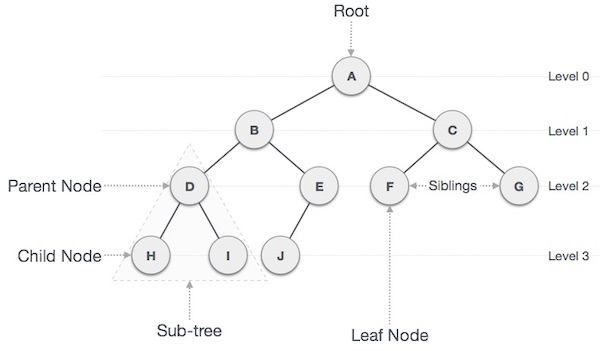# Graph

A graph data structure is a special type of nonlinear data structure which consists of a finite number of vertices or nodes, and edges or arcs. A graph can be both directed and undirected. A directed graph clearly indicates the direction of the edges, while an undirected graph mentions the edges, not the direction. As a result, in an undirected graph, both directions of edge are considered as a single edge. In other words, we can say a graph is a pair of sets (V, E), where V is the set of vertices and E is the set of edges:

V = {A, B, C, D, E, F}

E = {AB, BC, CE, ED, EF, DB}

In a directed graph, an edge AB is different from an edge BA while in an undirected graph, both AB and BA are the same. Graphs are handy to solve lots of complex problems in the programming world. We are going to continue our discussion of graph data structures in Chapter 9, Putting Graphs into Action. In the following diagram, we have: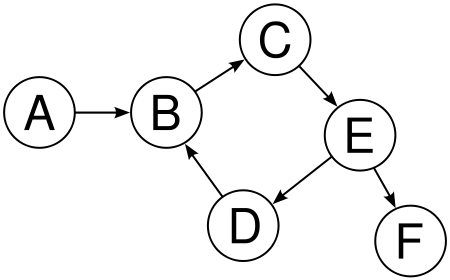# Heap

A heap is a special tree-based data structure which satisfies the heap properties. The largest key is the root and smaller keys are leaves, which is known as max heap. Or, the smallest key is the root and larger keys are leaves, which is known as min heap. Though the root of a heap structure is either the largest or smallest key of the tree, it is not necessarily a sorted structure. A heap is used for solving graph algorithms with efficiency and also in sorting. We are going to explore heap data structures in Chapter 10, Understanding and Using Heaps.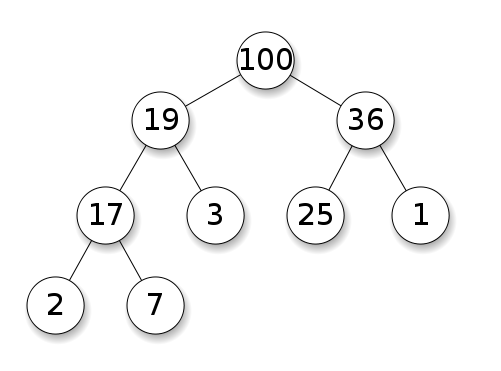# Solving a problem - algorithmic approach

So far we have discussed different types of data structures and their usage. But, one thing we have to remember is that just putting data in a proper structure might not solve our problems. We need to find solutions to our problems using the help of data structures or, in other words, we are going to solve problems using data structures. We need algorithms to solve our problem.

An algorithm is a step by step process which defines the set of instructions to be executed in a certain order to get a desired output. In general, algorithms are not limited to any programming language or platform. They are independent of programming languages. An algorithm must have the following characteristics:

• Input: An algorithm must have well-defined input. It can be 0 or more inputs.
• Output: An algorithm must have well-defined output. It must match the desired output.
• Precision: All steps are precisely defined.
• Finiteness: An algorithm must stop after a certain number of steps. It should not run indefinitely.
• Unambiguous: An algorithm should be clear and should not have any ambiguity in any of the steps.
• Independent: An algorithm should be independent of any programming language or platforms.

Let us now create an algorithm. But in order to do that, we need a problem statement. So let us assume that we have a new shipment of books for our library. There are 1000 books and they are not sorted in any particular order. We need to find books as per the list and store them in their designated shelves. How do we find them from the pile of books?

Now, we can solve the problem in different ways. Each way has a different approach to find out a solution for the problem. We call these approaches algorithms. To keep the discussion short and precise, we are going to only consider two approaches to solve the problem. We know there are several other ways as well but for simplicity let us keep our discussion only for one algorithm.

We are going to store the books in a simple row so that we can see the book names. Now, we will pick a book name from the list and search from one end of the row to the other end till we find the book. So basically, we are going to follow a sequential search for each of the books. We will repeat these steps until we place all books in their designated places.

# Writing pseudocode

Computer programs are written for machine reading. We have to write them in a certain format which will be compiled for the machine to understand. But often those written codes are not easy to follow for people other than programmers. In order to show those codes in an informal way so that humans can also understand, we prepare pseudocode. Though it is not an actual programming language code, pseudocode has similar structural conventions of a programming language. Since pseudocode does not run as a real program, there is no standard way of writing a pseudocode. We can follow our own way of writing a pseudocode.

Here is the pseudocode for our algorithm to find a book:

`Algorithm FindABook(L,book_name)   Input: list of Books L & name of the search book_name   Output: False if not found or position of the book we are looking for.   if L.size = 0 return null   found := false   for each item in L, do     if item = book_name, then       found := position of the item   return found `

Now, let us examine the pseudocode we have written. We are supplying a list of books and a name that we are searching. We are running a foreach loop to iterate each of the books and matching with the book name we are searching. If it is found, we are returning the position of the book where we found it, false otherwise. So, we have written a pseudocode to find a book name from our book list. But what about the other remaining books? How do we continue our search till all books are found and placed on the right shelf?:

`  Algorithm placeAllBooks     Input: list of Ordered Books OL, List of received books L     Output: nothing.     for each book_name in OL, do        if FindABook(L,book_name), then          remove the book from the list L          place it to the bookshelf `

Now we have the complete pseudocode for our algorithm of solving the book organization problem. Here, we are going through the list of ordered books and finding the book in the delivered section. If the book is found, we are removing it from the list and placing it to the right shelf.

This simple approach of writing pseudocode can help us solve more complex problems in a structured manner. Since pseudocodes are independent of programming languages and platforms, algorithms are expressed as pseudocode most of the time.

# Converting pseudocode to actual code

We are now going to convert our pseudocodes to actual PHP 7 codes as shown:

`function findABook(Array \$bookList, String \$bookName) {     \$found = FALSE;     foreach(\$bookList as \$index => \$book) {         if(\$book === \$bookName) {              \$found = \$index;              break;         }     }     return \$found; } function placeAllBooks(Array \$orderedBooks, Array &\$bookList) {     foreach (\$orderedBooks as \$book) {     \$bookFound = findABook(\$bookList, \$book);     if(\$bookFound !== FALSE) {         array_splice(\$bookList, \$bookFound, 1);     }   } } \$bookList = ['PHP','MySQL','PGSQL','Oracle','Java']; \$orderedBooks = ['MySQL','PGSQL','Java']; placeAllBooks(\$orderedBooks, \$bookList);echo implode(",", \$bookList); `

Let us now understand what is happening in the preceding code. First we have defined a new function, findABook at the beginning of the code. The function is defined with two parameters. One is Array \$bookList and the other is String \$bookName. At the beginning of the function we are initializing the \$found to FALSE, which means nothing has been found yet. The foreach loop iterates through the book list array \$bookList and for each book, it matches with our provided book name \$bookName. If the book name that we are looking for matches with the book in the \$bookList, we are assigning the index (where we found the match) to our \$found variable. Since we have found it, there is no point in continuing the loop. So, we have used the break command to get out of the loop. Just out of the loop we are returning our \$found variable. If the book was found, usually \$found will return any integer value greater than 0, else it will return false:

`function placeAllBooks(Array \$orderedBooks, Array &\$bookList) {     foreach (\$orderedBooks as \$book) {     \$bookFound = findABook(\$bookList, \$book);     if(\$bookFound !== FALSE) {         array_splice(\$bookList, \$bookFound, 1);     }   } } `

This particular function placeAllBooks actually iterates through our ordered books \$orderedBooks. We are iterating our ordered book list and searching each book in our delivered list using the findABook function. If the book is found in the ordered list (\$bookFound !== FALSE), we are removing that book from the delivered book list using the array_splice() function of PHP:

`\$bookList = ['PHP','MySQL','PGSQL','Oracle','Java'];\$orderedBooks = ['MySQL','PGSQL','Java'];`

These two lines actually shows two PHP arrays which are used for the list of books we have received, \$bookList and the list of books we have actually ordered \$orderedBooks. We are just using some dummy data to test our implemented code as shown:

`placeAllBooks(\$orderedBooks, \$bookList);`

The last part of our code actually calls the function placeAllBooks to perform the whole operation of checking each book searching for it in our received books and removing it, if it is in the list. So basically, we have implemented our pseudocode to an actual PHP code which we can use to solve our problem.

# Algorithm analysis

We have completed our algorithm in the previous section. But one thing we have not done yet is the analysis of our algorithm. A valid question in the current scenario can be, why do we really need to have an analysis of our algorithm? Though we have written the implementation, we are not sure about how many resources our written code will utilize. When we say resource, we mean both time and storage resource utilized by the running application. We write algorithms to work with any length of the input. In order to understand how our algorithm behaves when the input grows larger and how many resources have been utilized, we usually measure the efficiency of an algorithm by relating the input length to the number of steps (time complexity) or storage (space complexity). It is very important to do the analysis of algorithms in order to find the most efficient algorithm to solve the problem.

We can do algorithm analysis in two different stages. One is done before implementation and one after the implementation. The analysis we do before implementation is also known as theoretical analysis and we assume that other factors such as processing power and spaces are going to be constant. The after implementation analysis is known as empirical analysis of an algorithm which can vary from platform to platform or from language to language. In empirical analysis, we can get solid statistics from the system regarding time and space utilization.

For our algorithm to place the books and finding the books from purchased items, we can perform a similar analysis. At this time, we will be more concerned about the time complexity rather than the space complexity. We will explore space complexity in coming chapters.

# Calculating the complexity

There are two types of complexity we measure in algorithmic analysis:

• Time complexity: Time complexity is measured by the number of key operations in the algorithm. In other words, time complexity quantifies the amount of time taken by an algorithm from start to finish.
• Space complexity: Space complexity defines the amount of space (in memory) required by the algorithm in its life cycle. It depends on the choice of data structures and platforms.

Now let us focus on our implemented algorithm and find about the operations we are doing for the algorithm. In our placeAllBooks function, we are searching for each of our ordered books. So if we have 10 books, we are doing the search 10 times. If the number is 1000, we are doing the search 1000 times. So simply, we can say if there is n number of books, we are going to search it n number of times. In algorithm analysis, input number is mostly represented by n.

For each item in our ordered books, we are doing a search using the findABook function. Inside the function, we are again searching through each of the received books with a name we received from the placeAllBooks function. Now if we are lucky enough, we can find the name of the book at the beginning of the list of received books. In that case, we do not have to search the remaining items. But what if we are very unlucky and the book we are searching for is at the end of the list? We then have to search each of the books and, at the end, we find it. If the number of received books is also n, then we have to run the comparison n times.

If we assume that other operations are fixed, the only variable should be the input size. We can then define a boundary or mathematical equation to define the situation to calculate its runtime performance. We call it asymptotical analysis. Asymptotical analysis is input bound which means if there is no input, other factors are constant. We use asymptotical analysis to find out the best case, worst case, and average case scenario of algorithms:

• Best case: Best case indicates the minimum time required to execute the program. For our example algorithm, the best case can be that, for each book, we are only searching the first item. So, we end up searching for a very little amount of time. We use Ω notation (Sigma notation) to indicate the best case scenario.
• Average case: It indicates the average time required to execute a program. For our algorithm the average case will be finding the books around the middle of the list most of the time, or half of the time they are at the beginning of the list and the remaining half are at the end of the list.
• Worst case: It indicates the maximum running time for a program. The worst case example will be finding the books at the end of the list all the time. We use the O (big oh) notation to describe the worst case scenario. For each book searching in our algorithm, it can take O(n) running time. From now on, we will use this notation to express the complexity of our algorithm.

# Understanding the big O (big oh) notation

The big O notation is very important for the analysis of algorithms. We need to have a solid understanding of this notation and how to use this in the future. We are going to discuss the big O notation throughout this section.

Our algorithm for finding the books and placing them has n number of items. For the first book search, it will compare n number of books for the worst case situation. If we say time complexity is T, then for the first book the time complexity will be:

`T(1) = n`

As we are removing the founded book from the list, the size of the list is now n-1. For the second book search, it will compare n-1 number of books for the worst case situation. Then for the second book, the time complexity will be n-1. Combining the both time complexities, for first two books it will be:

`T(2) = n + (n - 1)`

If we continue like this, after the n-1 steps the last book search will only have 1 book left to compare. So, the total complexity will look like:

`T(n) = n + (n - 1) + (n - 2) + . . . . . . .  . . . . + 3 + 2 + 1 `

Now if we look at the preceding series, doesn't it look familiar? It is also known as the sum of n numbers equation as shown: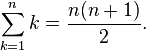So we can write:

`T(n) = n(n + 1)/2 `

Or:

`T(n) = n2/2 + n/2 `

For asymptotic analysis, we ignore low order terms and constant multipliers. Since we have n2, we can easily ignore the n here. Also, the 1/2 constant multiplier can also be ignored. Now we can express the time complexity with the big O notation as the order of n squared:

`T(n) = O(n2) `

Throughout the book, we will be using this big O notation to describe complexity of the algorithms or operations. Here are some common big O notations:

 Type Notation Constant O (1) Linear O (n) Logarithmic O (log n) n log n O (n log n) Quadratic O (n2) Cubic O (n3) Exponential O (2n)

# Standard PHP Library (SPL) and data structures

The Standard PHP Library (SPL), is one of the best possible features of the PHP language in last few years. SPL was created to solve common problems which were lacking in PHP. SPL extended the language in many ways but one of the striking features of SPL is its support of data structures. Though SPL is used for many other purposes, we are going to focus on the data structure part of SPL. SPL comes with core PHP installations and does not require any extension or change in configurations to enable it.

SPL provides a set of standard data structures through Object-Oriented Programming in PHP. The supported data structures are:

• Stack: It is implemented in SplStack by using SplDoublyLinkedList.
• Queue: It is implemented in SplQueue by using SplDoublyLinkedList.
• Heaps: It is implemented in SplHeap. It also supports max heap in SplMaxHeap and min heap in SplMinHeap.
• Priority queue: It is implemented in SplPriorityQueue by using SplHeap.
• Arrays: It is implemented in SplFixedArray for a fixed size array.
• Map: It is implemented in SplObjectStorage.

In coming chapters, we are going to explore each of the SPL data structure implementations and know their pros and cons, along with their performance analysis with our implementation of corresponding data structures. But as these data structures are already built in, we can use them for a quick turnaround of features and applications.

After the release of PHP 7, everyone was happy with the performance boost of the PHP application in general. PHP SPL is not having the similar performance boost in many cases, but we are going to analyze those in upcoming chapters.

# Summary

In this chapter, we have focused our discussion on basic data structures and their names. We have also learned about solving problems with defined steps, known as algorithms. We have also learned about analyzing the algorithms and the big O notation along with how to calculate the complexity. We had a simple brief about the built-in data structures in PHP in the form of SPL.

In the next chapter, we are going to focus on the PHP array, one of the most powerful, flexible data types in PHP. We are going to explore different uses of the PHP array to implement different data structures such as hash table, map, structs, and so on.